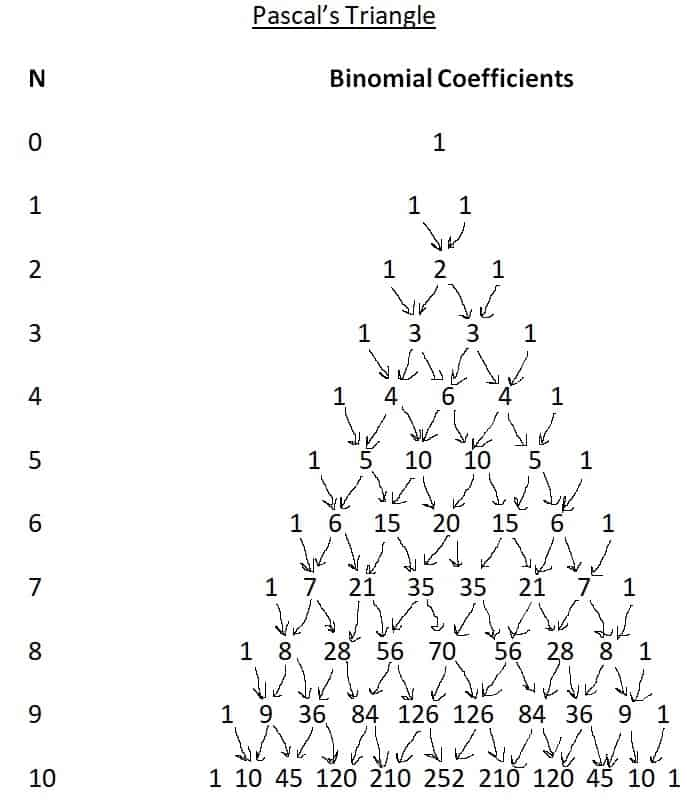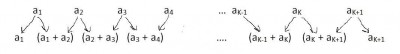# What Is Pascal’s Triangle? (10 Common Questions Answered)

Pascal’s Triangle is useful in algebra, probability, and combinatorics when we need to expand binomials raised to an integer power.  However, it always helps to know where it comes from and what it tells us.

So, what is Pascal’s Triangle?  Pascal’s Triangle is a triangle with rows that give us the binomial coefficients for the expansion of (x + 1)N. The top row of the triangle has one number, and the next row always has one more number that the previous row. The Nth row has (N + 1) entries, and the sum of these entries is 2N.

Of course, you can recreate Pascal’s Triangle easily from scratch if you know the first couple of rows and the algorithm to generate the next row.

In this article, we’ll talk about Pascal’s Triangle and what it can be used for.  We’ll also show some uses of Pascal’s Triangle in various scenarios.

Let’s get started.

## What Is Pascal’s Triangle?

Pascal’s Triangle is a triangle made up of rows of numbers which are binomial coefficients.  The top layer of the triangle has one number, and the next layer always has one more number than the previous layer.

If we give each row a natural number index, starting with N = 0 for the first row, then the nth row has N + 1 numbers in it.  The left and rightmost entries in a row are always 1.

The second and second to last numbers always have a value of N for N >= 1.

If we read a row from left to right, we get a number that is a palindrome (that is, it reads the same forwards and backwards).

### Why Is It Called Pascal’s Triangle?

Pascal’s Triangle is named after Blaise Pascal of France, who contributed to mathematics and other fields.  He wrote his “Treatise On The Arithmetical Triangle” in 1654, where he described his famous triangle which enumerates the binomial coefficients.

### How Do You Create Pascal’s Triangle?

To create Pascal’s triangle, begin with the lone number “1” in the first row (index N = 0).

Next, add a second row below it, with the number “1” appearing twice as separate entries (index N = 1).

Then, put a “1” at each end of the third row, and in the middle, add the two entries in the previous row (1 + 1 = 2) to get the entries “1  2  1” for the third row (index N = 2).

Continue to put a “1” at each end of each row.  In the middle, add pairs of consecutive entries from the previous row to fill out the next row.Each entry (besides the 1’s on the left and right) is the sum of a pair of entries from the previous row. For example, in the row for N = 5, the “10” is the sum of “4” and “6” from the previous row.

### What Is The Nth Row Of Pascal’s Triangle?

The Nth row of Pascal’s Triangle are given below for N = 0 to 9 (first through tenth rows):

• 1st row of Pascal’s Triangle: 1
• 2nd row of Pascal’s Triangle: 1, 1
• 3rd row of Pascal’s Triangle: 1, 2, 1
• 4th row of Pascal’s Triangle: 1, 3, 3, 1
• 5th row of Pascal’s Triangle: 1, 4, 6, 4, 1
• 6th row of Pascal’s Triangle: 1, 5, 10, 10, 5, 1
• 7th row of Pascal’s Triangle: 1, 6, 15, 20, 15, 6, 1
• 8th row of Pascal’s Triangle: 1, 7, 21, 35, 35, 21, 7, 1
• 9th row of Pascal’s Triangle: 1, 8, 28, 56, 70, 56, 28, 8, 1
• 10th row of Pascal’s Triangle: 1, 9, 36, 84, 126, 126, 84, 36, 9, 1

### Why Is Pascal’s Triangle Symmetrical?

Pascal’s Triangle is symmetrical because of the way it is constructed:

• Every row has a “1” at each end (so the first and last entries match up).
• Since the 2nd and 2nd to last entries in the previous row match (both are N – 1), the 2nd and 2nd to last entries in the next row will also  match (both will be N).
• The middle entries come from adding up consecutive pairs of entries in the previous row.  If the previous row is symmetrical, then the next row will be as well.  Since the first, second, and third rows are symmetrical, so will the fourth, fifth, sixth, etc.

### What Do The Numbers In Pascal’s Triangle Represent?

The numbers in Pascal’s Triangle represent binomial coefficients.  That is, they tell us the coefficients of terms after we expand (x + 1)n, combine like terms, and put the terms in descending order (xn comes first, and 1 comes last).

### How Does Pascal’s Triangle Relate To Binomial Expansion?

We can see that Pascal’s Triangle works for the first few values of n:

• (x + 1)0 = 1
• (x + 1)1 = x + 1
• (x + 1)2 = x2 + 2x + 1  [we can see this by using FOIL]
• (x + 1)3 = x3 + 3x2 + 3x + 1  [we can see this by multiplying the previous result by (x + 1)]

### What Can Pascal’s Triangle Be Used For?

In addition to binomial expansion in algebra, Pascal’s Triangle can also be used in probability and combinatorics.

#### Example Of Pascal’s Triangle In Probability

Let’s say that the probability of an event happening is p.  Then the probability of the complement (the event not happening) is 1 – p.

Let’s say we want to find the probability that n independent trials will all result in the event not happening.  In this case, we must calculate (1 – p)n, which is where Pascal’s Triangle will really come in handy!

Keep in mind that we must mind the negative signs, which alternate with even and odd coefficients.

For n = 6, Pascal’s Triangle gives us coefficients of  1, 6, 15, 20, 15, 6, 1:

• (1 – p)6 = 1 – 6p + 15p2 – 20p3 + 15p4 – 6p5 + p6

#### Example Of Pascal’s Triangle In Combinatorics

Let’s say that we have a license plate with A letters or numbers and B special characters.  If there are 8 spots on the license plate for letters, numbers, and characters, then we can find the total number of possible license plates by calculating (A + B)8.

For n = 8, Pascal’s Triangle gives us binomial coefficients of 1, 8, 28, 56, 70, 56, 28, 8, 1:

• (A + B)8 = A8 + 8A7B + 28A6B2 + 56A5B3 + 70A4B4 + 56A3B5 + 28A2B6 + 8AB7 + B8

### Is Pascal’s Triangle Infinite?

Pascal’s Triangle is infinite, since there is no limit to the number of rows that we can compute, and no limit to the whole number exponent N of a binomial expansion (x + y)N.

However, a given row of Pascal’s Triangle for a given value of N only has N + 1 entries, which is a finite number.

### Is Pascal’s Triangle A Fractal?

We can use Pascal’s Triangle to get an approximation of the Sierpinski triangle by coloring even numbers white and odd numbers black.Pascals Triangle with even numbers colored white and odd numbers colored black approximates a Sierpinski Triangle.

### How To Find The Sum Of A Row In Pascal’s Triangle

The sum of the Nth row of Pascal’s Triangle is 2N.  We can easily see this for the first few rows below:

• N = 0: sum = 1 = 20
• N = 1: sum = 1 + 1 = 2 = 21
• N = 2: sum = 1 + 2 + 1 = 4 = 22
• N = 3: sum = 1 + 3 + 3 + 1 = 8 = 23

We can show that this formula is true for all values of N with a proof by mathematical induction.

Base Case: we have already proven the result for N = 0, 1, 2, and 3 above.

Induction Step: assume the statement is true for N = K.  That is, assume that for the Kth row of Pascal’s Triangle, the entries have a sum of 2K.

This means that if the entries are a1, a2, a3, … , aK+1, then their sum is:

• a1 + a2 + a3 + … + aK+1 = 2K  [this is our Induction Hypothesis.  Note that a1 and aK+1 are both 1]

We want to prove that the sum of the (K + 1)st row is 2K+1.

We will simply follow the algorithm for constructing the (K + 1)st row from the Kth row.

First, we put a “1” at each end of the row.

Next, we add up pairs of entries from the Kth row to get the middle entries of the (K + 1)st row.

It might be easier to see how this works in the illustration below:We use each entry in the Kth row twice to get the entries in the (K + 1)st row of Pascal’s Triangle.

So, our entries in the (K + 1)st row will be:

• a1  [the first entry in the row, on the far left, which will always be 1]
• a1 + a2  [the second entry in the row]
• a2 + a3  [the third entry in the row]
• a3 + a4  [the fourth entry in the row]
• aK-1 + aK  [the third to last in the row]
• aK + aK+1  [the second to last entry in the row]
• aK+1  [the last entry in the row, on the far right, which will always be 1]

Adding up all of the entries in the (K + 1)st row gives us:

• a1 + (a1 + a2) + (a2 + a3) + (a3 + a4) + … + (aK-1 + aK) + (aK + aK+1) + aK+1
• =2a1 + 2a2 + 2a3 + 2a4 + … + 2aK-1 + 2aK + 2aK+1  [since each entry from the Kth row appears twice]
• =2[a1 + a2 + a3 + … + aK+1]  [factor out a 2]
• =2[2K]  [by the Induction Hypothesis]
• =2K+1

So, the (K + 1)st row of Pascal’s Triangle has a sum of 2K+1.  This finishes the proof by induction.